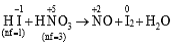# NEET Chemistry Redox Reactions Questions Solved

In the reaction:

XHI + YHNO3 $\to$NO + I2 + H2O

1. X=3, Y=2

2. X=2, Y=3

3. X=6, Y=2

4. X=6, Y=1

(3)Inverse of nf ratio is the mole ratio.

X:Y = 3:1 or 6:2

Difficulty Level:

• 14%
• 36%
• 46%
• 6%
Crack NEET with Online Course - Free Trial (Offer Valid Till August 24, 2019)